# Node.js MySQL Create Table

Introduction: Learn to create a table in MySQL database using NodeJS. We will see how to use the Create Table command in NodeJS using the MySQL module.

Prerequisite: Introduction to NodeJS MySQL

Setting up environment and Execution:

Step 1: Create a NodeJS Project and initialize it using the following command:

`npm init`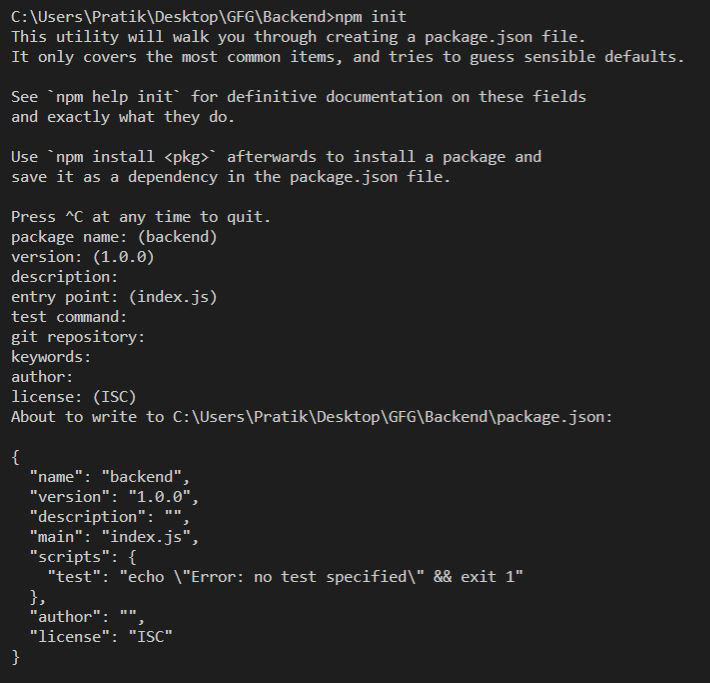Step 2: Install the express and mysql modules using the following command:

```npm install express
npm install mysql```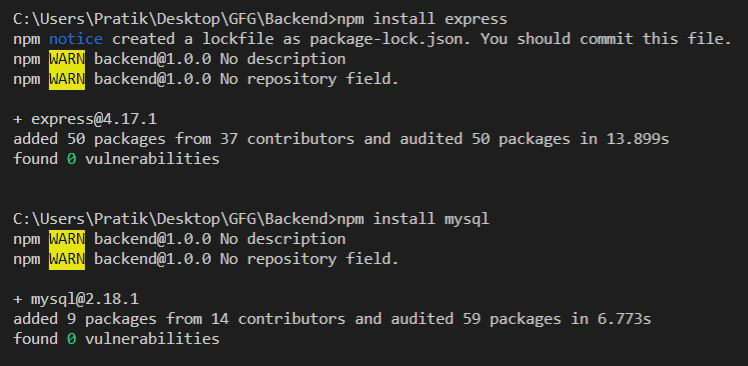File Structure: Our file structure will look like the following: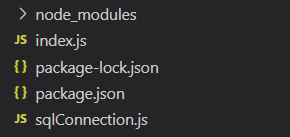Example: Create and export MySQL Connection Object as shown below:

Filename: sqlConnection.js

## Javascript

 `const mysql = require(``"mysql"``); ` ` `  `let db_con  = mysql.createConnection({ ` `    ``host: ``"localhost"``, ` `    ``user: ``"root"``, ` `    ``password: ``''``, ` `    ``database: ``'gfg_db'` `}); ` ` `  `db_con.connect((err) => { ` `    ``if` `(err) { ` `      ``console.log(``"Database Connection Failed !!!"``, err); ` `    ``} ``else` `{ ` `      ``console.log(``"connected to Database"``); ` `    ``} ` `}); ` ` `  `module.exports = db_con;`

Example 1: Creating a table without a primary key.

## index.js

 `const express = require(``"express"``); ` `const database = require(``'./sqlConnection'``); ` ` `  `const app = express(); ` ` `  `app.get(``"/"``, (req, res) => { ` `   `  `  ``let tableName = ``'gfg_table'``; ` ` `  `  ``// Query to create table ` `  ``let query = `CREATE TABLE \${tableName} ( ` `    ``name VARCHAR(255), address VARCHAR(255))`; ` ` `  `  ``database.query(query, (err, rows) => { ` `      ``if``(err) ``return` `res.status(500) ` `          ``.send(``"Table Creation Failed"``); ` ` `  `      ``return` `res.send( ` ``Successfully Created Table - \${tableName}`); ` `  ``}) ` `}); ` ` `  `app.listen(5000, () => { ` `  ``console.log(`Server is up and running on 5000 ...`); ` `});`

Run the index.js file using the following command:

`node index.js`

Output:

`Successfully Created Table - gfg_table`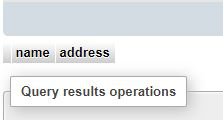Example 2: Create a Table with an auto-increment primary key.

## index.js

 `const express = require(``"express"``); ` `const database = require(``'./sqlConnection'``); ` ` `  `const app = express(); ` ` `  `app.get(``"/"``, (req, res) => { ` `     `  `    ``let tableName = ``'gfg_table'``; ` ` `  `    ``// Query to create table ` `    ``let query = `CREATE TABLE \${tableName}  ` `        ``(id INT AUTO_INCREMENT PRIMARY KEY,  ` `        ``name VARCHAR(255), address VARCHAR(255))`; ` ` `  `    ``database.query(query, (err, rows) => { ` `        ``if``(err) ``return` `res.status(500) ` `            ``.send(``"Table Creation Failed"``); ` ` `  `        ``return` `res.send( ` ``Successfully Created Table - \${tableName}`); ` `    ``}) ` `}); ` ` `  `app.listen(5000, () => { ` `  ``console.log(`Server is up and running on 5000 ...`); ` `}); `

Run the index.js file using the following command:

`node index.js`

Output:

`Successfully Created Table - gfg_table`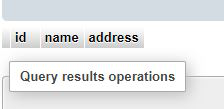Whether you're preparing for your first job interview or aiming to upskill in this ever-evolving tech landscape, GeeksforGeeks Courses are your key to success. We provide top-quality content at affordable prices, all geared towards accelerating your growth in a time-bound manner. Join the millions we've already empowered, and we're here to do the same for you. Don't miss out - check it out now!

Previous
Next